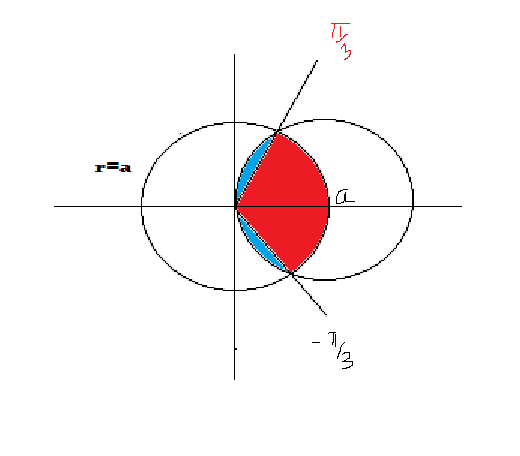Coffee Room
Discuss anything here - everything that you wish to discuss with fellow engineers.
12838 Members
Join this group to post and comment.RVignesh • Jan 30, 2012

# mathematics area of curve

plz help me to find the common area of following two curves
R=a
R=2acos@
This is eqn of circle in polar form. I tried but couldnt figure out the solutionsimplycoder • Jan 30, 2012RVignesh • Jan 30, 2012
In polar form put r=under root x2+y2 and tan@ is y/x
so the required eqn are
x2+ y2=a2
(x-a)2+y2=a2
so now we can draw two circles
the required area will be 2(area of upper curve -lower curve)
the upper curve is second circle nd lower curve is first one.
I took Limit of integrtn as 0 to a/2
but I dont get the answer.RVignesh • Jan 30, 2012
I changed the limit for half the curve frm 0 to a/2 and then frm a/2 to asimplycoder • Jan 31, 2012
I will give you a hint here, draw the figure on paper and see whether you have correct limits.RVignesh • Jan 31, 2012
I did thatsimplycoder • Jan 31, 2012
Can you put a pic that might show how did you get those limits?
How did you come with limits from a/2 to a and other stuff?
What is the center of the two circles. If you can get this right then only we are on correct path.
Did you try to find the second integral limits?
What co-ordinate system did you choose? what is the second integral?

Think about it conceptually and not something to solve and match the answer.
BTW if you have the final answer, then post that too, so that when I finally explain there wouldnt be any issues as there are multiple equivalent forms of same answer.RVignesh • Jan 31, 2012
as the equation is in polar form so first convert it to cartesian co ordinates . As i have explained previously. So the centres comes out to be 0,0 and a,0. And radius for both are a . Now when we draw both circles intersect on x axis . So that point is a/2 ,0.
Now you tell me what to do now.
This is gates question. Answer is 1.77 a2.I_am_Kunal :) • Jan 31, 2012
RVignesh
as the equation is in polar form so first convert it to cartesian co ordinates . As i have explained previously. So the centres comes out to be 0,0 and a,0. And radius for both are a . Now when we draw both circles intersect on x axis . So that point is a/2 ,0.
Now you tell me what to do now.
This is gates question. Answer is 1.77 a2.
The circles do not intersect on the x-axis. You are right about the centers. But you should recheck the points of intersections. The circles intersect at two points. X co-ordinate of both of which is a/2. Y co-ordinate is not 0.simplycoder • Jan 31, 2012
Since we are interested in polar co-ordinates, we only want to know more in terms of @ and a.
So you got a (Though I think its incorrect) Now search for limits in terms of @.

Then double integrate, still if you dont get, I shall upload the answer tomorrow.

Also I donot agree with your answer and so if there is any method along it, post that aswell. I am getting 1.228(a^2). How?? Will let you know by tomorrow.simplycoder • Feb 1, 2012
This is a rough sketch of what your graph should look like.
Solve for @ and you get that it intersects in two points pi/3 and -pi/3.
The red area is from (@=-pi/3 to pi/3) and (r=0 to a).
The blue area follows the r=2cos@ circle.
And there are two such of them. So no integrate
I(r=0 to a , @=-pi/3 to pi/3)rdrd@ ................................(1)
2*I(r=0 to cos2@ , @=pi/3 to pi/2)rdrd@ ................................(2)